Get instant live expert help with Excel or Google Sheets“My Excelchat expert helped me in less than 20 minutes, saving me what would have been 5 hours of work!”

#### Post your problem and you’ll get expert help in seconds.

Your message must be at least 40 characters
Our professional experts are available now. Your privacy is guaranteed.

# How Do You Create a Random Schedule in Excel?

Excel allows us to create a random schedule using the RAND, INDEX, RANK.EQ and COUNTIF functions. This step by step tutorial will assist all levels of Excel users to get the random weekly task list.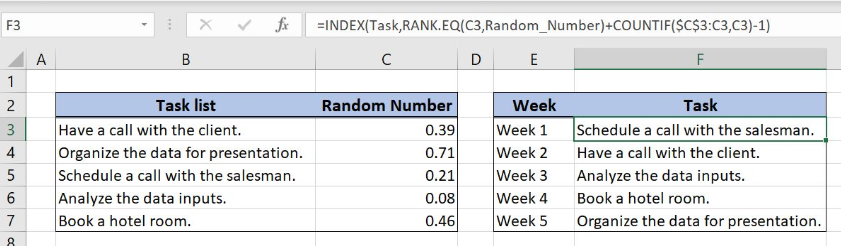Figure 1. Create a random schedule in Excel

## Syntax of the RAND Formula

The generic formula for the RAND function is:

`=RAND()`

The function returns a random decimal number between 0 and 1 and has no parameters.

## Syntax of the INDEX Formula

The generic formula for the INDEX function is:

`=INDEX(array, row_num, column_num)`

The parameters of the INDEX function are:

• array – a range of cells where we want to get a data
• row_num – a number of a row in the array for which we want to get a value
• column_num – a column in the array which returns a value.

## Syntax of the RANK.EQ Formula

The generic formula for the RANK.EQ function is:

`=RANK.EQ(number, ref, [order])`

The parameters of the RANK.EQ function are:

• number – a number for which we want to find the rank in the array
• ref – the array where we want to rank the number
• [order] – a type of ranking data in the ascending or the descending order (0 or omitted value).

## Syntax of the COUNTIF Formula

The generic formula for the COUNTIF function is:

`=COUNTIF(range, criteria)`

The parameters of the COUNTIF function are:

• range – the range from which we want to count the non-blank cells
• criteria – the criteria for counting the cells in the range

## Setting up Our Data to Create a Random Schedule in Excel

Our first table consists of 2 columns: “Task list” (column B) and “Random Number” (column C). The second table consists of 2 columns: “Week” (column E) and “Task” (column F). The idea is to randomly fill column F with the tasks from column B.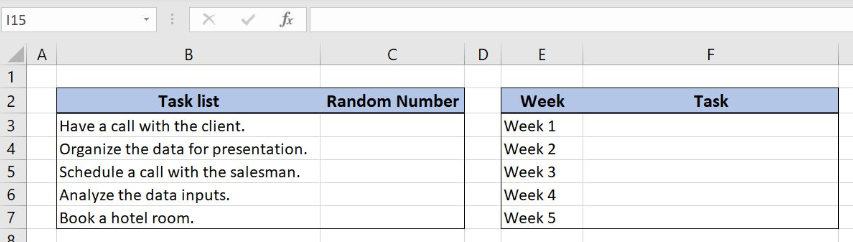Figure 2. Data for the random schedule

## Create a Random Schedule with RAND, INDEX, RANK.EQ and COUNTIF Functions

We want to get the random task from column B and to place the result in column F. In order to make the formula more clear, we will create a named range Task for cell range B3:B7 and a named range Random_Number for the cell range C3:C7.

To create a named range we should follow the steps:

• Select the cell range that should be named
• Click on the name box in Excel
• Write the name for the cell range and press enter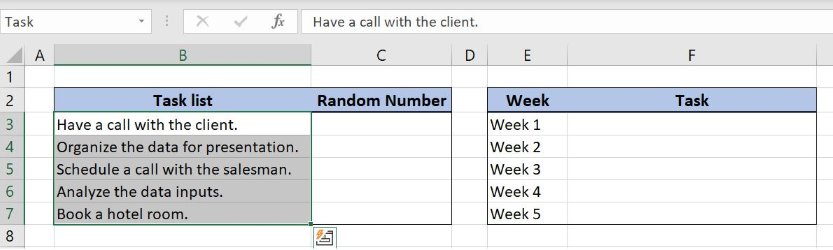Figure 3. Creating a named range Task for column “Task list”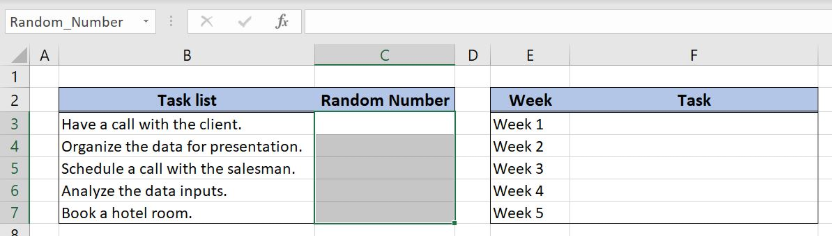Figure 4. Creating a named range Random_Number for column “Random Number”

The formula in Column C looks like:

`=RAND()`

The RAND function gets the random value from 0 to 1. The randomized values are placed in column C and will help us to get the random schedule in column F.

To apply the formula, we need to follow these steps:

• Select cell C3 and click on it
• Insert the formula: `=RAND()`
• Press enter.
• Drag the formula down to the other cells in the column by clicking and dragging the little “+” icon at the bottom-right of the cell.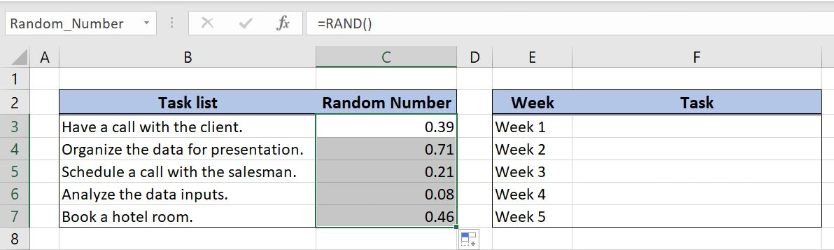Figure 5. Using the RAND formula to get a random value between 0 and 1

Now we can place the formula in the cell F3 to get the weekly random task list

`=INDEX(Task,RANK.EQ(C3,Random_Number)+COUNTIF(\$C\$3:C3,C3)-1)`

The parameter array in the INDEX function is a named range Task while the row_num is the formula RANK.EQ(C3,Random_Number)+COUNTIF(\$C\$3:C3,C3)-1. In the RANK.EQ function, the number is a cell C3 while the parameter ref is the named range Random_Number. An order is omitted because we want to rank in descending order. Finally, the parameter range in the COUNTIF function is the cell range \$C\$3:C3 while the criteria is the cell C3.

To apply the formula, we need to follow these steps:

• Select cell F3 and click on it
• Insert the formula: `=INDEX(Task,RANK.EQ(C3,Random_Number)+COUNTIF(\$C\$3:C3,C3)-1)`
• Press enter.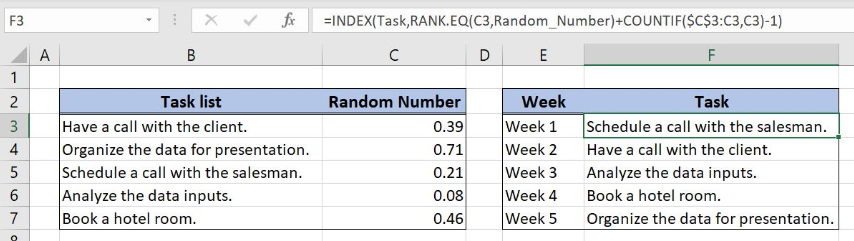Figure 6. Get the random schedule with RAND, INDEX, RANK.EQ and COUNTIF functions

The RANK.EQ function result is number 3, the rank of the cell C3 in column C. The COUNTIF function checks if the value in the cell C3 appears for the first time in the list. Since the value in the cell C3 is a unique value COUNTIF formula result is number 1. After this step INDEX function looks like: INDEX(\$B\$3:\$B\$7,3+1-1)  and final formula result is the 3rd task from the column “Task list”.

Most of the time, the problem you will need to solve will be more complex than a simple application of a formula or function. If you want to save hours of research and frustration, try our live Excelchat service! Our Excel Experts are available 24/7 to answer any Excel question you may have. We guarantee a connection within 30 seconds and a customized solution within 20 minutes.

Solution examplesI need a formula to count the number of rows that contain at least one of two possible phrases, but not give me the total number of instances that both phrases occur overall.
Solved by E. B. in 33 minsI need help on index and match where the return is not correct
Solved by B. B. in 20 minsI have a database extract that is showing the same clients multiple times in the list. How can I write a formula in excel to look at the column with the client names and return only unique records on another sheet? I want to run this over several files so wanting to avoid using pivot tables which is how I would normal do this.
Solved by X. J. in 20 minsi have a table with Dates, First names , Last names and i want to count how many times a name occurs, but if a name occurs more than once on a particular date i need to only count it once. this is on excel and not google sheets
Solved by F. H. in 31 minsNeed a formula that counts (1,2,3,4 ect..in cell M21) a range of cells, N4:N20, which are annual premium values. But I only want to add a sale count if the average monthly premium is above \$30 per sale. So if someone has 4 sales but they don't equal a total of \$120/mth (\$30 x 4 = \$120)... they would only get a count of 3 sales.
Solved by S. E. in 60 mins